Difference between revisions of "Cole equation of state"

The Cole equation of state  is the adiabatic version of the stiffened equation of state for liquids. (See Derivation, below.) It has the form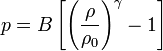$p = B \left[ \left( \frac{\rho}{\rho_0} \right)^\gamma -1 \right]$

In it,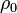$\rho_0$ is a reference density around which the density varies,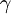$\gamma$ is the adiabatic index, and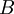$B$ is a pressure parameter.

Usually, the equation is used to model a nearly incompressible system. In this case, the exponent is often set to a value of 7, and$B$ is large, in the following sense. The fluctuations of the density are related to the speed of sound as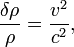$\frac{\delta \rho}{\rho} = \frac{v^2}{c^2} ,$

where$v$ is the largest velocity, and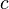$c$ is the speed of sound (the ratio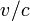$v/c$ is Mach's number). The speed of sound can be seen to be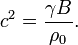$c^2 = \frac{\gamma B}{\rho_0}.$

Therefore, if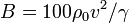$B=100 \rho_0 v^2 / \gamma$, the relative density fluctuations will be about 0.01.

If the fluctuations in the density are indeed small, the equation of state may be approximated by the simpler: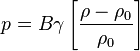$p = B \gamma \left[ \frac{\rho-\rho_0}{\rho_0} \right]$

It is quite common that the name "Tait equation of state" is improperly used for this EOS. This perhaps stems for the classic text by Cole calling this equation a "modified Tait equation" (p. 39).

Derivation

Let us write the stiffened EOS as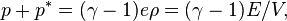$p+ p^* = (\gamma -1) e \rho = (\gamma -1) E / V ,$

where E is the internal energy. In an adiabatic process, the work is the only responsible of a change in internal energy. Hence the first law reads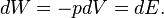$dW= -p dV = dE .$

Taking differences on the EOS,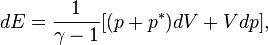$dE = \frac{1}{\gamma-1} [(p+p^*) dV + V dp ] ,$

so that the first law can be simplified to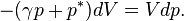$- (\gamma p + p^*) dV = V dp.$

This equation can be solved in the standard way, with the result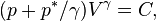$( p + p^* / \gamma) V^\gamma = C ,$

where C is a constant of integration. This derivation closely follows the standard derivation of the adiabatic law of an ideal gas, and it reduces to it if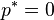$p^* =0$.

If the values of the thermodynamic variables are known at some reference state, we may write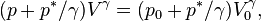$( p + p^* / \gamma) V^\gamma = ( p_0 + p^* / \gamma) V_0^\gamma ,$

which can be written as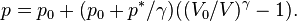$p = p_0 + ( p_0 + p^* / \gamma) ( (V_0/V)^\gamma - 1 ) .$

Going back to densities, instead of volumes,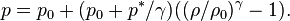$p = p_0 + ( p_0 + p^* / \gamma) ( (\rho/\rho_0)^\gamma - 1) .$

Comparing with the Cole EOS, we can readily identify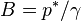$B = p^* / \gamma$

Moreover, the Cole EOS differs slightly, as it should read (as indeed does in e.g. the book by Courant)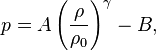$p = A \left( \frac{\rho}{\rho_0} \right)^\gamma - B ,$

with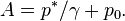$A = p^* / \gamma + p_0 .$

This difference is negligible for liquids but for an ideal gas$p^*=0$ and there is a huge difference, B being zero and A being equal to the reference pressure.

Now, the speed of sound is given by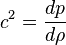$c^2=\frac{dp}{d\rho}$

with the derivative taken along an adiabatic line. This is precisely our case, and we readily obtain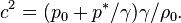$c^2= ( p_0 + p^* / \gamma) \gamma /\rho_0 .$

From this expression a value of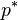$p^*$ can be deduced. For water,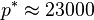$p^*\approx 23000$ bar, from which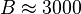$B\approx 3000$ bar. If the speed of sound is used in the EOS one obtains the rather elegant expression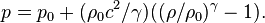$p = p_0 + ( \rho_0 c^2 / \gamma) ( (\rho/\rho_0)^\gamma - 1) .$